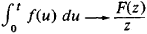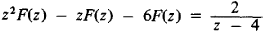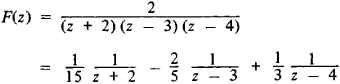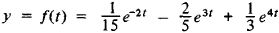# Operational Calculus

Also found in: Wikipedia.

## operational calculus

[‚äp·ə′rā·shən·əl ′kal·kyə·ləs]
(mathematics)
A technique by which problems in analysis, in particular differential equations, are transformed into algebraic problems, usually the problem of solving a polynomial equation. Also known as operational analysis.
McGraw-Hill Dictionary of Scientific & Technical Terms, 6E, Copyright © 2003 by The McGraw-Hill Companies, Inc.
The following article is from The Great Soviet Encyclopedia (1979). It might be outdated or ideologically biased.

## Operational Calculus

a method of mathematical analysis that in a number of cases permits the solution of complicated mathematical problems by means of simple rules. It is particularly important in such fields as mechanics, automation, and electrical engineering.

Operational calculus essentially involves the replacement of functions under study by other functions called transforms, which are obtained from the original functions by certain rules. Usually the transform is obtained from the original function through a Laplace transformation. In the substitution, the differential operator p = d/dt is interpreted as an algebraic quantity, with the result that the integration of certain classes of linear differential equations and the solution of a number of other problems of mathematical analysis reduce to the solution of simpler algebraic problems. Thus, the solution of a linear differential equation reduces to the generally simpler problem of solving an algebraic equation; from the algebraic equation one finds the transform of the solution of the original equation, and then the solution itself is recovered from the transform. The operation of finding the transform of the original function and the reverse operation are facilitated by the existence of extensive tables of transforms.

The work of the British scientist O. Heaviside was very important for the development of operational calculus. Heaviside set forth formal rules for dealing with the operator p = d/dt and certain functions of this operator. By means of operational calculus he solved a number of very important problems in electrodynamics. He did not, however, supply a mathematical grounding for operational calculus, and many of his results remained unproved.

A rigorous foundation for operational calculus was provided with the aid of the Laplace integral transform. If the function f(t), 0 ≤ t < + ∞, is transformed into the function F(z), z = x+ iy.

f(t)→ F(z)

then the derivative

(*) fʹ →zF(z)–f(0)

and the integralThus, the differential operator p is transformed into an operator of multiplication by the variable z, and integration reduces to division by z. Table 1 gives some examples, for t ≥ 0, of transforms.

Table 1
Original functionTransform
f(t) F(z)
1 1/Z
tn n!lzn+1 (n > 0, an integer)
eλt 1/(z–λ)
COS ωt z/(z2 + ω2)
sin ωt ω/(z2 + ω2)

Example. With the aid of operational calculus we shall find the solution y = f(t) of the linear differential equation

yʹʹ – yʹ–6y = 2e4t

given with the initial conditions

y0 = f(0) = 0 and yʹ0 = fʹ(0)= 0

Using Table 1, we move from the unknown function f(t) and the given function 2e4t to the transforms F(z) and 2/(z —4). Applying formula (*) for the transforms of the derivatives, we obtainorHence, again using Table 1,In 1953 the Polish mathematician J. Mikusiński suggested that operational calculus could be based on the concept of a

function ring. The theory of generalized functions can also be used as a foundation for the methods of operational calculus. There are various generalizations of operational calculus, such as multidimensional operational calculus, which is based on the theory of multiple integrals. An operational calculus of differential operators other than the operator p = d/dt has been created, for example, B = (d/dt)t(d/dt). This theory is also based on the study of function rings, in which the concept of the product of functions is defined in a suitable manner.

### REFERENCES

Ditkin, V. A., and A. P. Prudnikov. Spravochnik po operatsionnomu ischisleniiu. Moscow, 1965.
Ditkin, V. A., and A.P. Prudnikov. Operatsionnoe ischislenie. Moscow, 1966.
Mikusiński, J. Operatsionnoe ischislenie. Moscow, 1956. (Translated from Polish.)
Shtokalo, I. Z. Operatsionnoe ischislenie. Kiev, 1972.

V. A. DITKIN

References in periodicals archive ?
Associated with a scalar type spectral operator in a complex Banach space is the Borel operational calculus analogous to that for a normal operator in a complex Hilbert space [6, 7, 9, 10], which assigns to any Borel measurable function F : [sigma](A) [right arrow] C a scalar type spectral operator
The properties of the spectral measure and operational calculus are exhaustively delineated in [6, 7].
are understood in the sense of the Borel operational calculus (see, e.g., [9, 10]) and the function T(*) is replaceable with any nonnegative, continuous, and increasing on [0, [infinity]) function F(*) satisfying
There is also symbolism tied to a city or parts of a city that are magnified beyond their tactical value, drawing exertions by either side disproportionate to a strict operational calculus. Identifying and understanding these elements present a complex problem for intelligence collection and analysis.
Prudnikov, Reference Book on Operational Calculus, Higher School, Moscow, Russia, 1965 (Russian).
McLachlan introduces modern operational calculus based on the Laplace transform to graduate engineers and technologists, though he suggests that the purely mathematical part of his treatment may be useful to advanced undergraduates in mathematics.
They divide the calculus and distribute it to their successors (neighboring nodes with one hop), which forms progressively and dynamically a calculus diffusion tree, where only the leaf nodes (Workers) in the tree which does operational calculus. The inner nodes divide and conquer tasks (Masters).
The third scenario is one with which many are already familiar owing to the West's well-known if excessively paranoid fears of Pakistan becoming a failed state a gigantic Yugoslavia like entity with hundreds of nukes and legions of militant obscurantists determined to build a jihadist utopia guided by an operational calculus incompatible with the logic of deterrence and interest based statecraft.
His topics include quotient spaces, the uniform boundedness principle, projections, the Fredholm alternative, symmetric linear operators, sesquilinear functional, and an operational calculus.
Prudnikov (1927-99) introduce operational calculus and the related topics of integral transforms of functions and generalized functions.
Associated with a scalar type spectral operator in a complex Banach space is the Borel operational calculus analogous to that for a normal operator in a complex Hilbert space [7, 8,10,11], which assigns to any Borel measurable function F : [sigma](A) [right arrow] C a scalar type spectral operator
The properties of the spectral measure and operational calculus, exhaustively delineated in [7, 8], underlie the entire subsequent discourse.

Site: Follow: Share:
Open / Close